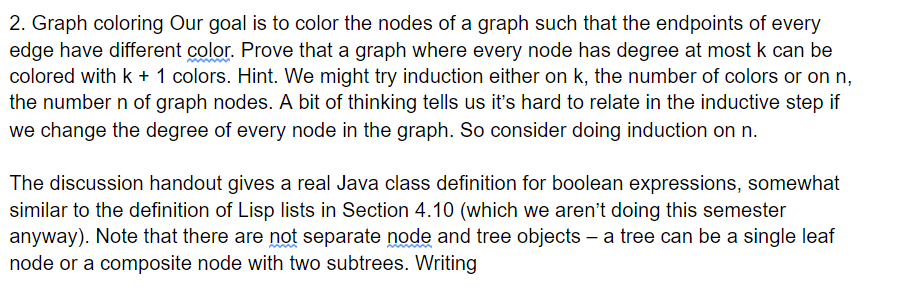# Question Solved1 Answer2. Graph coloring Our goal is to color the nodes of a graph such that the endpoints of every edge have different color. Prove that a graph where every node has degree at most k can be colored with k + 1 colors. Hint. We might try induction either on k, the number of colors or on n, the number n of graph nodes. A bit of thinking tells us it's hard to relate in the inductive step if we change the degree of every node in the graph. So consider doing induction on n. The discussion handout gives a real Java class definition for boolean expressions, somewhat similar to the definition of Lisp lists in Section 4.10 (which we aren't doing this semester anyway). Note that there are not separate node and tree objects - a tree can be a single leaf node or a composite node with two subtrees. WritingFQSNWJ The Asker · Computer ScienceTranscribed Image Text: 2. Graph coloring Our goal is to color the nodes of a graph such that the endpoints of every edge have different color. Prove that a graph where every node has degree at most k can be colored with k + 1 colors. Hint. We might try induction either on k, the number of colors or on n, the number n of graph nodes. A bit of thinking tells us it's hard to relate in the inductive step if we change the degree of every node in the graph. So consider doing induction on n. The discussion handout gives a real Java class definition for boolean expressions, somewhat similar to the definition of Lisp lists in Section 4.10 (which we aren't doing this semester anyway). Note that there are not separate node and tree objects - a tree can be a single leaf node or a composite node with two subtrees. Writing
More
Transcribed Image Text: 2. Graph coloring Our goal is to color the nodes of a graph such that the endpoints of every edge have different color. Prove that a graph where every node has degree at most k can be colored with k + 1 colors. Hint. We might try induction either on k, the number of colors or on n, the number n of graph nodes. A bit of thinking tells us it's hard to relate in the inductive step if we change the degree of every node in the graph. So consider doing induction on n. The discussion handout gives a real Java class definition for boolean expressions, somewhat similar to the definition of Lisp lists in Section 4.10 (which we aren't doing this semester anyway). Note that there are not separate node and tree objects - a tree can be a single leaf node or a composite node with two subtrees. Writing